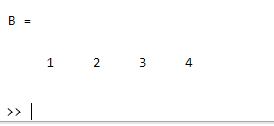Open In App

# How to Remove Nan Values from a Matrix in MATLAB?

Removal of Nan Values from a Matrix.There are multiple methods by which we can remove Nan values from a specified matrix:.

### Method 1: By using rmmissing( )

This function is used to remove missing entries or Nan values from a specified matrix.

### Syntax

`rmmissing(A)`

Parameters: This function accepts a parameter which is illustrated below:

• A: This is the specified matrix of elements.

Return Value: It returns the matrix without missing entries or Nan values.

Example:

## Matlab

 `% MATLAB code for remove NaN ``% values using rmmissing()``A = [1, NaN, 2, 3 NaN, 4]; ``% Initializing``% of matrix `` ` `% Calling the rmmissing() function over``% the above matrix``B = rmmissing(A)`

Output:### Method 2: By using isnan( )

This function is used to return a matrix of 1 i.e. true for the elements like NaN, and 0 i.e. false when they are not.

### Syntax

`isnan(A)`

Parameters: This function accepts a parameter.

A: This is the specified matrix of elements.

Return Value: It returns the matrix of logical values.

Example:

## Matlab

 `% MATLAB code for remove NaN values using isnan()``A = [1, 2, NaN, 3, 4]; ``% Initializing of matrix`` ` `% Calling isnan() function and getting matrix``% boolean values i.e. 1 or 0``B = isnan(A)`` ` `% Getting a new matrix "C" without NaN values``% which was originally "A"``C = A(~B)`

Output: